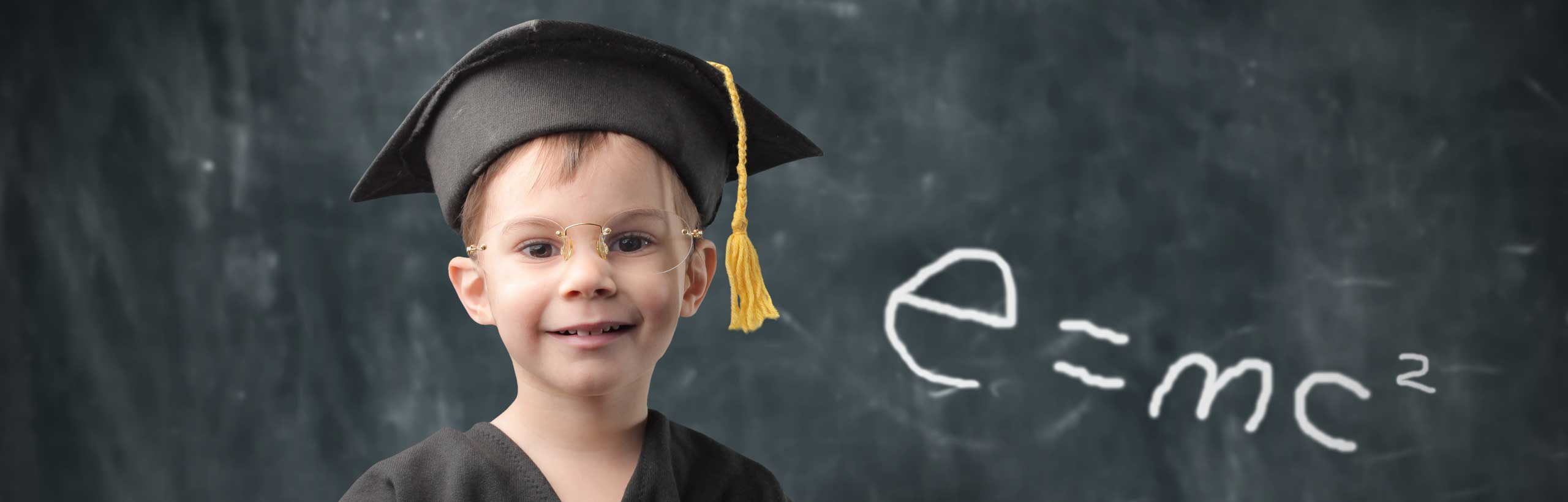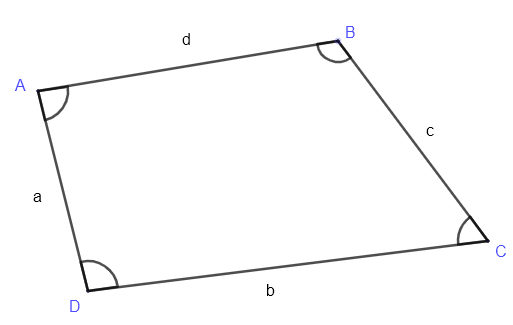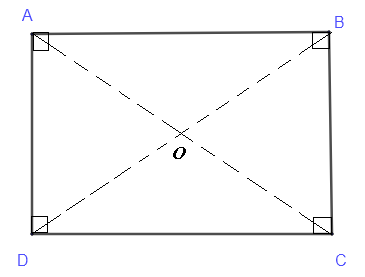A quadrilateral $ABCD$ is a closed shape with four sides (edges) and four vertices. It is also called a polygon with four sides.The four interior angles of a quadrilateral add up to $360^{\circ}$, hence $\quad \angle A + \angle B + \angle C + \angle D = 360^{\circ}$
Special quadrilaterals such as parallelograms, trpaezoids, rectangles, squares, kites and rhombuses are presented with their properties.

## Parallelogram

A parallelogram is a quadilateral with the opposite sides parallel.Properties

1. Opposite sides are congruent (equal length)
2. The diagonals $AC$ and $BD$ bisects the at point $O$ that divides each diagonal into equal parts: $OA = OC$ and $OB = OD$
3. The are several pairs of congruent triangles: $\triangle ADB$ and $\triangle CBD$ , $\triangle ADC$ and $\triangle ABC$ , $\triangle ADO$ and $\triangle BCO$ etc ...
4. Opposite interior angles are congruent: $\angle DAB = \angle DCB$ and $\angle ADC = \angle ABC$
5. Several other angles are congruent: $\angle ABO = \angle CDO$, $\angle DAO = \angle BCO$, etc ...
6. Area $A_p$ of a parallelogram is given by: $A_r = AB \times h$
Parallelogram Problems and Calculator

## Trapezoid

A trapezoid is a quadilateral with at least two opposite sides parallel.Area $A_t$ of a trapezoid is given by: $A_t = \dfrac{1}{2} h \times (AB+DC)$
Trapezoid Problems and Calculator

## RectangleProperties
A rectangle is a parallelogram with interior angles equal to $90^{\circ}$. Hence all properties of parallelograms are also property of rectangles and more properties are listed below.

1. $OA = OB = OC = OD$
2. $\triangle ABC$, $\triangle BCD$, etc ... are rigth triangles
3. Area $A_{re}$ of a rectangle is given by: $A_{re} = AB \times BC$
Rectangle Problems and Calculator

## Square

A square is a rectangle with all sides equal.Properties
All properties of rectangles are also property of squares and more properties are listed below.

1. The diagonals intersect at angle of $90^{\circ}$
2. Area $A_{s}$ of a rectangle is given by: $A_{s} = AB^2$
Problems on Squares and Calculator

## Kite

A kite is a quadrilateral with two pairs of adjacent sides equal in length. In the diagram below, sides $BA$ and $BC$ have equal lengths and sides $DA$ and $DC$ have equal lengths.Properties

1. The diagonals $AC$ and $BD$ bisects the at point $O$ at an angle of $90^{\circ}$.
2. The diagonals $AC$ and $BD$ bisects the at point $O$ that divides the diagonal $AC$ into equal parts: $OA = OC$
3. $BOA$, $BOC$, $DOA$ and $DOC$ are right triangles.
4. $\angle ABO = \angle CBO$, $\angle ADO = \angle CDO$, etc ...
5. Triangles $BOA$ and $BOC$ are congruent, etc ...
6. Area $A_k$ of a kite is given by: $A_k = \dfrac{1}{2} \times AC \times BD$
Kite Questions with Solutions and Calculator

## Rhombus

A rhombus is a parallelogram will all 4 sides equal in length.Properties

1. The diagonals $AC$ and $BD$ bisects the at point $O$ at an angle of $90^{\circ}$.
2. The diagonals $AC$ and $BD$ bisects the at point $O$ that divides the two diagonals into equal parts: $OA = OC$ and $OD = OB$
3. $BOA$, $BOC$, $DOA$ and $DOC$ are congruent triangles.
4. $\angle ABO = \angle CBO$, $\angle ADO = \angle CDO$, etc ...
5. Area $A_{ro}$ of a parallelogram is given by: $A_{ro} = \dfrac{1}{2} \times AC \times BD$
Rhombus Problems and Calculator